# C++11右值引用：移动语义和完美转发

## 指针成员和拷贝构造函数

#include <iostream>
using namespace std;

class HasPtrMem
{
public:
HasPtrMem():d(new int(0))
{}
///拷贝构造函数，从堆中分配内存，并用*h.d初始化
//HasptrMem(const HasPtrMem& h):d(new int(*h.d))
//{}

~HasPtrMem()
{
delete d;
}
public:
int* d;
};

int main ()
{
HasPtrMem a;
HasPtrMem b(a);
cout << a.d << endl;
cout << b.d << endl;
return 0;
}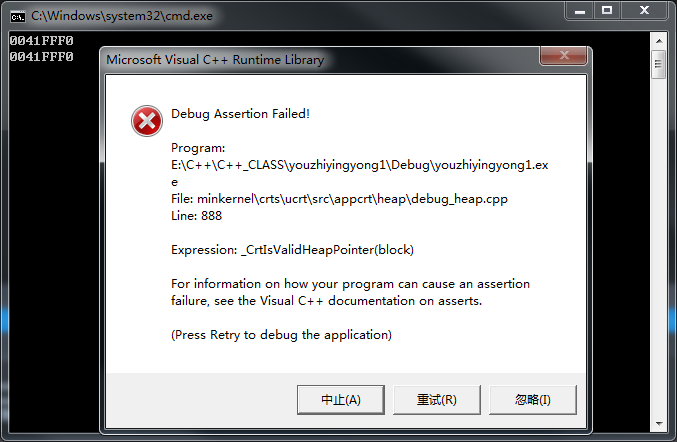#include <iostream>
using namespace std;

class HasPtrMem
{
public:
HasPtrMem():d(new int(0))
{}
///拷贝构造函数，从堆中分配内存，并用*h.d初始化
HasPtrMem(const HasPtrMem& h):d(new int(*h.d))
{}

~HasPtrMem()
{
delete d;
}
public:
int* d;
};



## 左值、右值与右值引用

a = b + c;
a是左值，b+c是一个右值。通过&a可以获取该变量的地址；表达式b+c、函数int func()的返回值是右值，在其被赋值给某一变量前，我们不能通过变量名找到它，＆(b+c)这样的操作则不会通过编译。

• 左值
可以取地址的、有名字的，是代表一个内存地址值，并且通过这个内存地址，就可以对内存进行读并且写（主要是能写）操作；

• 右值
不能取地址的、没有名字的，是代表一个常量或者是与运算操作符结合的表达式，简单点就是一个数据值。右值又分为:

• 纯右值（prvalue，Pure Rvalue）: 纯右值的概念等同于我们在C++98标准中右值的概念，指的是临时变量和不跟对象关联的字面量值。包括：
• 1、非引用返回的函数返回的临时变量值
• 2、运算表达式，如1+3产生的临时变量值
• 3、不跟对象关联的字面量，如2、’c‘、true
• 4、类型转换函数的返回值
• 5、lamda表达式
• 将亡值（xvalue，eXpiring Value）:C++11新增的跟右值引用相关的表达式，这样表达式通常是将要被移动的对象（移为他用），将亡值可以理解为通过“盗取”其他变量内存空间的方式获取到的值。包括：
• 返回右值引用T&&的函数返回值
• std::move的返回值
• 转换为T&&的类型转换函数的返回值
• 左值引用和右值引用
右值引用和左值引用都是属于引用类型。左值引用就是对一个左值进行引用的类型。右值引用就是对一个右值进行引用的类型。无论是声明一个左值引用还是右值引用，都必须立即进行初始化。而其原因可以理解为是引用类型本身自己并不拥有所绑定对象的内存，只是该对象的一个别名。左值引用是具名变量值的别名，而右值引用则是不具名（匿名）变量的别名。
举例说明：
T&& a = ReturnRvalue();
ReturnRvalue函数返回的右值在表达式语句结束后，其生命也就终结了，而通过右值引用的声明，该右值又“重获新生”，其生命期将于右值引用类型a的生命期一样。只要a还“活着”，该右值临时量将会一直“存活”下去。
T b= ReturnRvalue();
右值引用的声明方式会少一次对象的析构和一次对象构造。因为a是右值引用，直接绑定了ReturnRvalue()返回的临时量，而b是由临时值构造的，而临时量在表达式结束后会析构因而会多一次析构和构造的开销。
注意：
能够声明右值引用a的前提是ReturnRvalue返回的是一个右值。通常右值引用是不能够绑定到任何左值的，如下代码会导致编译无法通过：

int c;
int &&d = c;


1） 右值值引用通常不能绑定到任何的左值，要想绑定一个左值到右值引用，通常需要std::move()将左值强制转换为右值。
2）左值引用通常也不能绑定到右值，但常量左值引用是个“万能”的引用类型。它可以接受非常量左值、常量左值、右值对其进行初始化。不过常量左值所引用的右值在它的“余生”中只能是只读的。相对地，非常量左值只能接受非常量左值对其进行初始化。

const bool & judgement = true;

const bool judgement = true;

T ReturnRvalue() {}
T& e = ReturnRvalue();          // 编译失败
const T& f = ReturnRvalue();    // 编译通过
T&& g = ReturnRvalue();         // 编译通过


#include <iostream>
using namespace std;

struct Copyable {
Copyable() {}
Copyable(const Copyable &o) {
cout << "Copied" << endl;
}
};

Copyable ReturnRvalue() { return Copyable(); }
void AcceptVal(Copyable) {}
void AcceptRef(const Copyable & ) {}

int main() {
cout << "Pass by value: " << endl;
AcceptVal(ReturnRvalue()); // 临时值被拷贝传入
cout << "Pass by reference: " << endl;
AcceptRef(ReturnRvalue()); // 临时值被作为引用传递
}


1、Copyable结构体的唯一的作用就是在被拷贝构造的时候打印：Copied。
2、AcceptVal使用了值传递参数，而AcceptRef使用了引用传递。
3、在以ReturnRvalue返回的右值为参数的时 候，AcceptRef就可以直接使用产生的临时值（并延长其生命期），而AcceptVal则不能直接使用临时对象。

Pass by value:
Copied
Copied
Pass by reference:
Copied


const T && crvalueref = ReturnRvalue();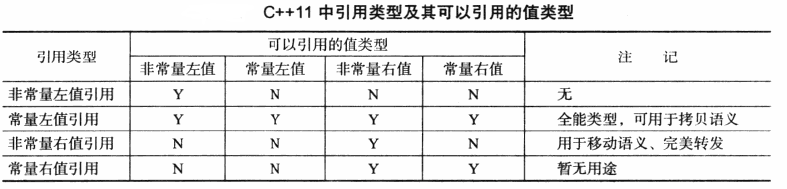①Object(T&);       //复制构造，仅接受左值
②Object(const T&); //复制构造，即可以接受左值又可接收右值
③Object(T&&) noexcept; //移动构造，仅接受右值
④T& operator=(const T&);//复制赋值函数，即可以接受左值又可接收右值
⑤T& operator=(T&&); //移动赋值函数，仅接受右值


## std::move 强制转化为右值

static_cast<T&&>（Ivalue）

#include <iostream>
class Moveable {
public:
Moveable(): i(new int(3)) {}
~Moveable() { delete i; }
Moveable(const Moveable& m):　i(new int(*m.i)) {}
Moveable(Moveable&& m): i(m.i) { m.i = nullptr; }
int* i;
};
int main() {
Moveable a;
Moveable c(move(a));    // 调用移动构造函数
cout << *a.i << endl;   // 运行错误
}



Moveable c（move（a）;
a本来是个左值变量，通过 std: move将其转换为右值。这样一来，a.i就被c的移动构造函数设置为指针空值。由于a的生命期实际要到main函数结束才结束，那么随后对表达式*a.i进行计算的时候，就会发生严重的运行时错误。

#include <iostream>
using namespace std;

class HugeMem
{
public:
HugeMem(int size) : sz(size > 0 ? size : 1)
{
c = new int[sz];
}
~HugeMem()
{
delete []c;
}
HugeMem(HugeMem && hm) : sz(hm.sz), c(hm.c)
{
hm.c = nullptr;
}

int* c;
int sz;
};

class Moveable
{
public:
Moveable() : i(new int(3)), h(1024) { }
~Moveable() { delete i; }
Moveable(Moveable && m) : i(m.i), h(move(m.h)) // 强制转为右值，以调用移动构造函数
{
m.i = nullptr;
}

int* i;
HugeMem h;
};

Moveable GetTemp()
{
Moveable tmp = Moveable();
cout << hex << "Huge Mem from " << __func__ << " @" << tmp. h. c << endl; // Huge Mem from GetTemp @0x0086E490
return tmp;
}

int main()
{
Moveable a(GetTemp());
cout << hex << "Huge Mem from " << __func__ << " @" << a. h. c << endl; // Huge Mem from main @0x0086E490
}


1、定义了两个类型：HugeMem和Moveable，其中Moveable包含了一个HugeMem的对象。
2、在Moveable的移动构造函数中，std::move函数将m.h强制转化为右值，以迫使Moveable中的h能够实现移动构造。
3、这里可以使用std::move，是因为m.h是m的成员，既然m将在表达式结束后被析构，其成员也自然会被析构，因此不存在生存期不合理的问题。

4、如果不使用std::move(m.h)这样的表达式，而是直接使用m.h这个表达式，由于m.h是个左值，就会导致调用HugeMem的拷贝构造函数来构造Moveable的成员h。移动语义就没有能够成功地向类的成员传递。换言之，还是会由于拷贝而导致一定的性能上的损失。

## 移动语义

#include <iostream>
using namespace std;

class HasPtrMem {
public:
HasPtrMem() : d(new int(0)) {
cout << "Construct: " << ++n_cstr << endl;
}
HasPtrMem(const HasPtrMem& h) : d(new int(*h.d)) {
cout << "Copy construct: " << ++n_cptr << endl;
}
~HasPtrMem() {
cout << "Destruct: " << ++n_dstr << endl;
}
int *d;
static int n_cstr ;
static int n_cptr ;
static int n_dstr ;
};
int HasPtrMem::n_cstr = 0;
int HasPtrMem::n_cptr = 0;
int HasPtrMem::n_dstr = 0;

HasPtrMem getTemp()
{
return  HasPtrMem();
}

int main() {
HasPtrMem a = getTemp();
//return 0;
}


VS2015编译结果：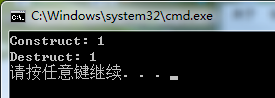ubuntu18.04 编译

g++ test.cpp -o test -fno-elide-constructors
./test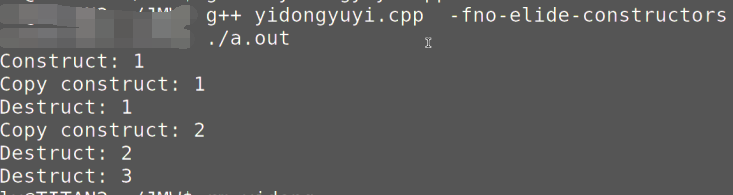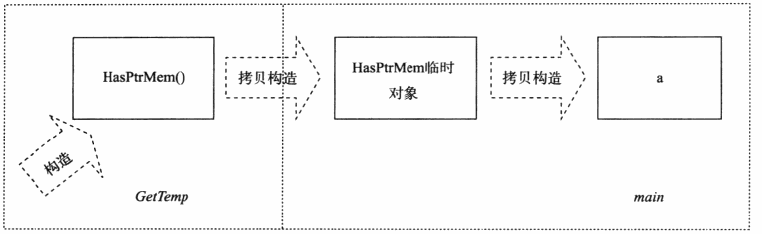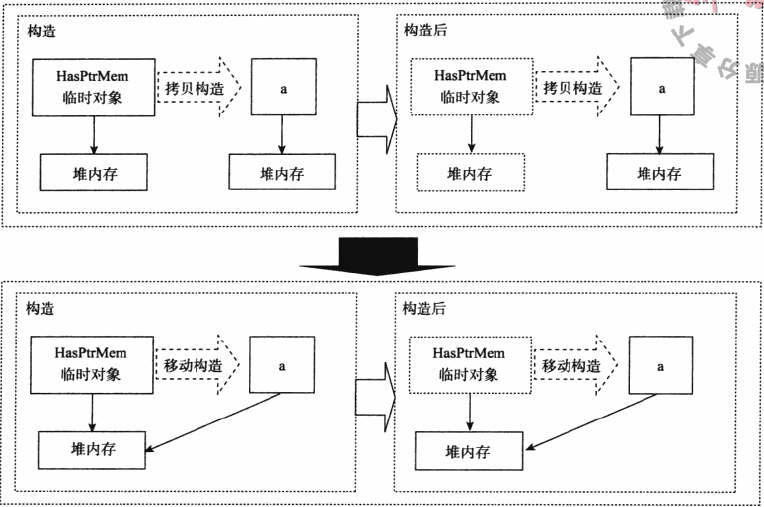#include <iostream>
using namespace std;

class HasPtrMem
{
public:
HasPtrMem() : d(new int(3))
{
cout << "Construct: " << ++n_cstr << endl;
}

HasPtrMem(const HasPtrMem & h) : d(new int(*h.d))
{
cout << "Copy construct: " << ++n_cptr << endl;
}

HasPtrMem(HasPtrMem && h) : d(h.d)    // 移动构造函数
{
h.d = nullptr;                  // 将临时值的指针成员置空
cout << "Move construct: " << ++n_mvtr << endl;
}

~HasPtrMem()
{
delete d;
cout << "Destruct: " << ++n_dstr << endl;
}

int * d;
static int n_cstr;
static int n_dstr;
static int n_cptr;
static int n_mvtr;
};
int HasPtrMem::n_cstr = 0;
int HasPtrMem::n_cptr = 0;
int HasPtrMem::n_mvtr = 0;
int HasPtrMem::n_dstr = 0;

HasPtrMem GetTemp() {
return HasPtrMem();
}

int main() {
HasPtrMem m = GetTemp();
return 0;
}



Has PtrMem类多了一个构造函数 HasPtrMen（ HasPtrMem&&），这个就是我们所谓的移动构造函数。与拷贝构造函数不同的是，移动构造

Construct:1
Move construct:1
Destruct:1
Move construct:2
Destruct:2
Destruct:3


### 移动语义其他问题

1. 移动语义一定是要改变临时变量的值
Moveable(const Moveable &&);
const Moveable ReturnVal();


1. 在C++11中，拷贝/移动改造函数有以下3个版本：
T Object(T&)
T Object(const T&)
T Object(T&&)


1. 实现移动语义，则表明该类型的变量拥有的资源只能被移动，不能被拷贝，那么这样的资源必须是唯一的，如智能指针、文件流。

2. 尽量不要编写会抛出异常的移动构造函数，因为有可能移动没完成，会导致一些指针成为悬挂指针，通过添加noexcept关键字，可以保证移动构造函数抛出异常直接终止程序。

### 移动语义举例

#include <iostream>
#include <cstring>
#include <vector>
using namespace std;

class MyString
{
public:
static size_t CCtor; //统计调用拷贝构造函数的次数
//    static size_t CCtor; //统计调用拷贝构造函数的次数
public:
// 构造函数
MyString(const char* cstr=0){
if (cstr) {
m_data = new char[strlen(cstr)+1];
strcpy(m_data, cstr);
}
else {
m_data = new char;
*m_data = '\0';
}
}

// 拷贝构造函数
MyString(const MyString& str) {
CCtor ++;
m_data = new char[ strlen(str.m_data) + 1 ];
strcpy(m_data, str.m_data);
}
// 拷贝赋值函数 =号重载
MyString& operator=(const MyString& str){
if (this == &str) // 避免自我赋值!!
return *this;

delete[] m_data;
m_data = new char[ strlen(str.m_data) + 1 ];
strcpy(m_data, str.m_data);
return *this;
}

~MyString() {
delete[] m_data;
}

char* get_c_str() const { return m_data; }
private:
char* m_data;
};
size_t MyString::CCtor = 0;

int main()
{
vector<MyString> vecStr;
vecStr.reserve(1000); //先分配好1000个空间，不这么做，调用的次数可能远大于1000
for(int i=0;i<1000;i++){
vecStr.push_back(MyString("hello"));
}
cout << MyString::CCtor << endl;
}


#include <iostream>
#include <cstring>
#include <vector>
using namespace std;

class MyString
{
public:
static size_t CCtor; //统计调用拷贝构造函数的次数
static size_t MCtor; //统计调用移动构造函数的次数
static size_t CAsgn; //统计调用拷贝赋值函数的次数
static size_t MAsgn; //统计调用移动赋值函数的次数

public:
// 构造函数
MyString(const char* cstr=0){
if (cstr) {
m_data = new char[strlen(cstr)+1];
strcpy(m_data, cstr);
}
else {
m_data = new char;
*m_data = '\0';
}
}

// 拷贝构造函数
MyString(const MyString& str) {
CCtor ++;
m_data = new char[ strlen(str.m_data) + 1 ];
strcpy(m_data, str.m_data);
}
// 移动构造函数
MyString(MyString&& str) noexcept
:m_data(str.m_data) {
MCtor ++;
str.m_data = nullptr; //不再指向之前的资源了
}

// 拷贝赋值函数 =号重载
MyString& operator=(const MyString& str){
CAsgn ++;
if (this == &str) // 避免自我赋值!!
return *this;

delete[] m_data;
m_data = new char[ strlen(str.m_data) + 1 ];
strcpy(m_data, str.m_data);
return *this;
}

// 移动赋值函数 =号重载
MyString& operator=(MyString&& str) noexcept{
MAsgn ++;
if (this == &str) // 避免自我赋值!!
return *this;

delete[] m_data;
m_data = str.m_data;
str.m_data = nullptr; //不再指向之前的资源了
return *this;
}

~MyString() {
delete[] m_data;
}

char* get_c_str() const { return m_data; }
private:
char* m_data;
};
size_t MyString::CCtor = 0;
size_t MyString::MCtor = 0;
size_t MyString::CAsgn = 0;
size_t MyString::MAsgn = 0;
int main()
{
vector<MyString> vecStr;
vecStr.reserve(1000); //先分配好1000个空间
for(int i=0;i<1000;i++){
vecStr.push_back(MyString("hello"));
}
cout << "CCtor = " << MyString::CCtor << endl;
cout << "MCtor = " << MyString::MCtor << endl;
cout << "CAsgn = " << MyString::CAsgn << endl;
cout << "MAsgn = " << MyString::MAsgn << endl;
}

/* 结果
CCtor = 0
MCtor = 1000
CAsgn = 0
MAsgn = 0
*/



int main()
{
vector<MyString> vecStr;
vecStr.reserve(1000); //先分配好1000个空间
for(int i=0;i<1000;i++){
MyString tmp("hello");
vecStr.push_back(tmp); //调用的是拷贝构造函数
}
cout << "CCtor = " << MyString::CCtor << endl;
cout << "MCtor = " << MyString::MCtor << endl;
cout << "CAsgn = " << MyString::CAsgn << endl;
cout << "MAsgn = " << MyString::MAsgn << endl;

cout << endl;
MyString::CCtor = 0;
MyString::MCtor = 0;
MyString::CAsgn = 0;
MyString::MAsgn = 0;
vector<MyString> vecStr2;
vecStr2.reserve(1000); //先分配好1000个空间
for(int i=0;i<1000;i++){
MyString tmp("hello");
vecStr2.push_back(std::move(tmp)); //调用的是移动构造函数
}
cout << "CCtor = " << MyString::CCtor << endl;
cout << "MCtor = " << MyString::MCtor << endl;
cout << "CAsgn = " << MyString::CAsgn << endl;
cout << "MAsgn = " << MyString::MAsgn << endl;
}

/* 运行结果
CCtor = 1000
MCtor = 0
CAsgn = 0
MAsgn = 0

CCtor = 0
MCtor = 1000
CAsgn = 0
MAsgn = 0
*/


MyString str1("hello"); //调用构造函数
MyString str2("world"); //调用构造函数
MyString str3(str1); //调用拷贝构造函数
MyString str4(std::move(str1)); // 调用移动构造函数、
//    cout << str1.get_c_str() << endl; // 此时str1的内部指针已经失效了！不要使用
//注意：虽然str1中的m_dat已经称为了空，但是str1这个对象还活着，知道出了它的作用域才会析构！而不是move完了立刻析构
MyString str5;
str5 = str2; //调用拷贝赋值函数
MyString str6;
str6 = std::move(str2); // str2的内容也失效了，不要再使用



1、str6 = std::move(str2)，虽然将str2的资源给了str6，但是str2并没有立刻析构，只有在str2离开了自己的作用域的时候才会析构，所以，如果继续使用str2的m_data变量，可能会发生意想不到的错误。
2、如果我们没有提供移动构造函数，只提供了拷贝构造函数，std::move()会失效但是不会发生错误，因为编译器找不到移动构造函数就去寻找拷贝构造函数，也这是拷贝构造函数的参数是const T&常量左值引用的原因！
3、c++11中的所有容器都实现了move语义，move只是转移了资源的控制权，本质上是将左值强制转化为右值使用，以用于移动拷贝或赋值，避免对含有资源的对象发生无谓的拷贝。move对于拥有如内存、文件句柄等资源的成员的对象有效，如果是一些基本类型，如int和char数组等，如果使用move，仍会发生拷贝（因为没有对应的移动构造函数），所以说move对含有资源的对象说更有意义。

## 完美转发

template <typename T>
void IamForwarding（T t） {
IrunCodeActually(t);
}


IamForwording是一个转发函数模板。而函数 Irun Code Actually则是真正执行代码的目标函数。这是一个参数透传的实现，但是因为使用最基本类型转发，会在传参的时候产生一次额外的临时对象拷贝，因为只能说是转发，但不完美。

void IrunCodeActually(int t) {}
template <typename T>
void IamForwarding（const T& t） {
IrunCodeActually(t);
}


C++11是通过引入一条所谓 “引用折叠”（ reference collapsing） 的新语言规则，并结合新的模板推导规则来完成完美转发。

typedef const A T;
typedef T& TR;
TR& v = 1;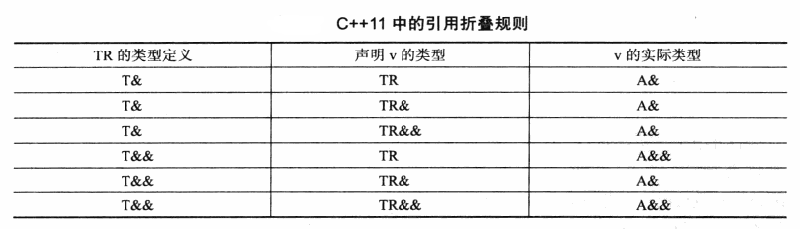template <typename T>
void IamForwarding（T&& t） {
IrunCodeActually(static_cast<T&&>(t));
}


void IamForwarding（X& && t） {
IrunCodeActually(static_cast<X& &&>(t));//将X& 看成T
}


void IamForwarding（X& t） {
IrunCodeActually(static_cast<X&>(t));
}


IrunCodeActually如果接受左值引用的话，就可以直接调用转发函数。这里的 static cast是留给传递右值用的。

void IamForwarding（X&& && t） {
IrunCodeActually(static_cast<X&& &&>(t));
}


void IamForwarding（X&& t） {
IrunCodeActually(static_cast<X&&>(t));
}


template <typename T>
void IamForwarding（T&& t） {
IrunCodeActually(forward(t));
}


move和forward实现差别不大，但是为了不同用途，有了不同命名。

#include <iostream>

using namespace std;

void run(int && m) { cout << "rvalue ref" << endl; }
void run(int & m) { cout << "lvalue ref" << endl; }
void run(const int && m) { cout << "const rvalue ref" << endl; }
void run(const int & m) { cout << "const lvalue ref" << endl; }

template <typename T>
void perfectForward(T&& t) {
run(forward<T>(t));
}

int main(){
int a;
int b;
const int c = 1;
const int d = 0;

perfectForward(a);
perfectForward(move(b));
perfectForward(c);
perfectForward(move(d));

return 0;
}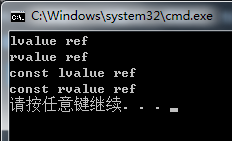## 总结

• 由两种值类型，左值和右值。
• 有三种引用类型，左值引用、右值引用和通用引用。左值引用只能绑定左值，右值引用只能绑定右值，通用引用由初始化时绑定的值的类型确定。
• 左值和右值是独立于他们的类型的，右值引用可能是左值可能是右值，如果这个右值引用已经被命名了，他就是左值。
• 引用折叠规则：所有的右值引用叠加到右值引用上仍然是一个右值引用，其他引用折叠都为左值引用。当T&&为模板参数时，输入左值，它将变成左值引用，输入右值则变成具名的右值应用。
• 移动语义可以减少无谓的内存拷贝，要想实现移动语义，需要实现移动构造函数和移动赋值函数。
• std::move()将一个左值转换成一个右值，强制使用移动拷贝和赋值函数，这个函数本身并没有对这个左值什么特殊操作。

## 参考

1、深入理解C++11：C++11新特性解析与应用
2、https://www.jianshu.com/p/d19fc8447eaa

02-10122
07-0286709-253564
10-09253
10-113419
12-2562
06-173974
08-1675
10-051万+
05-101346